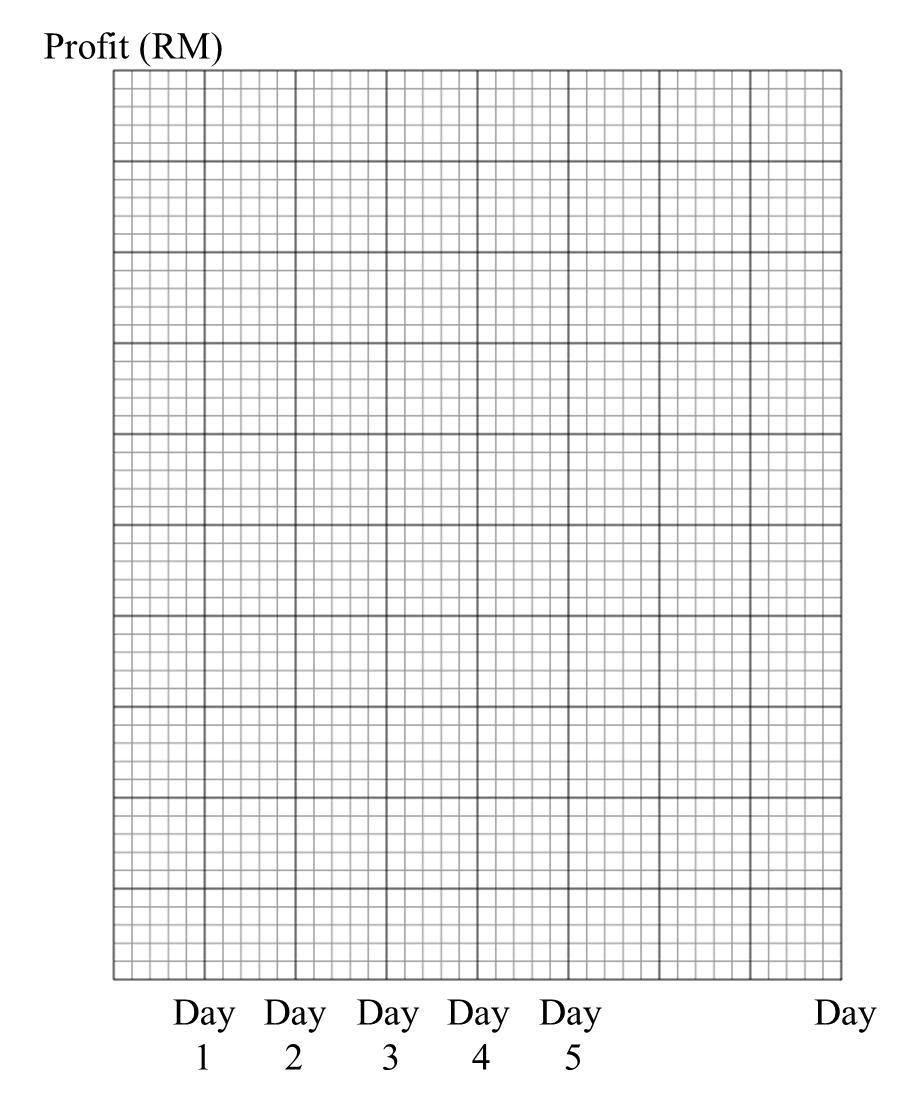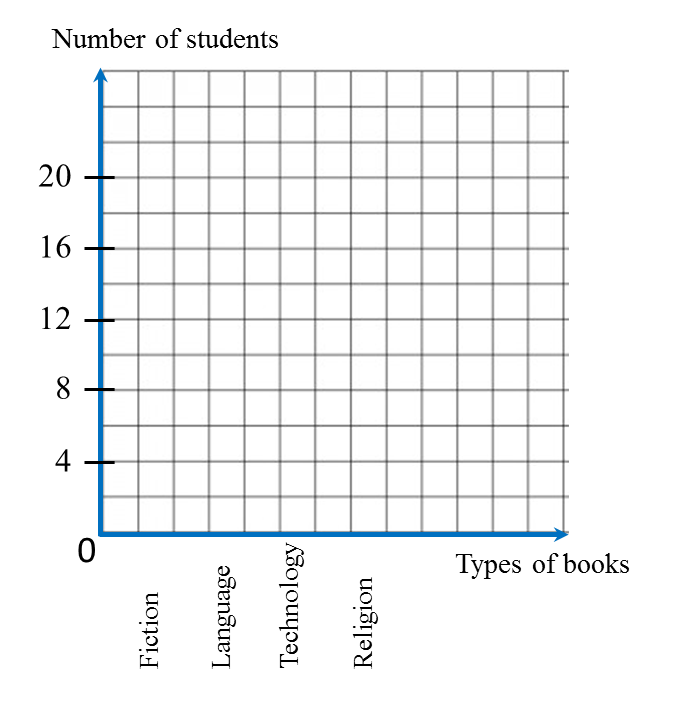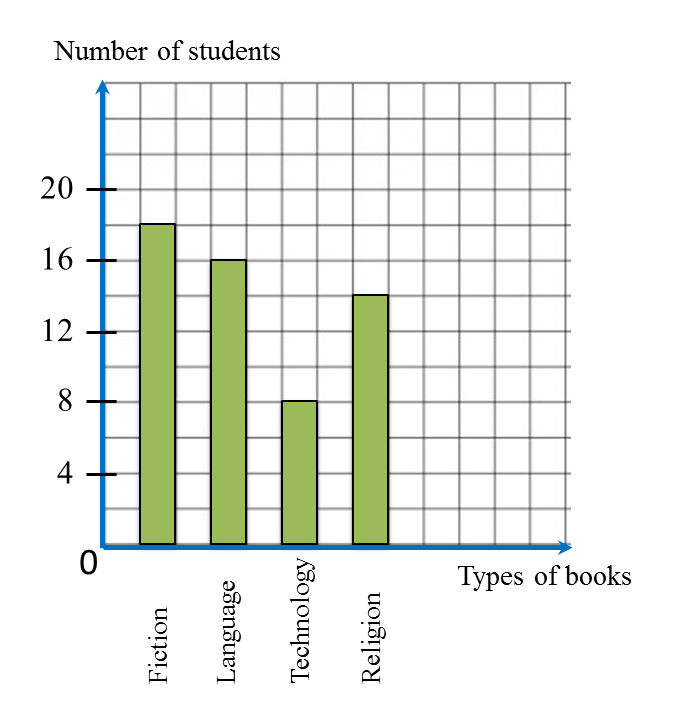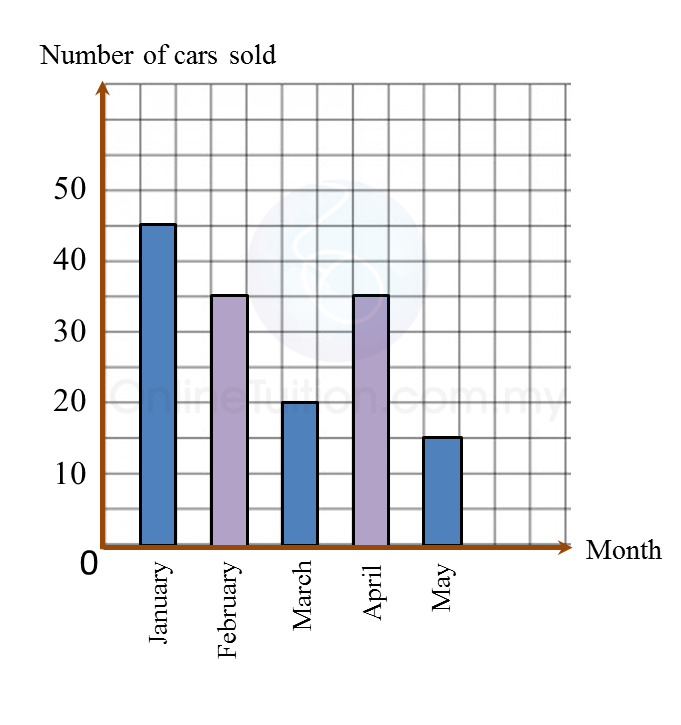# 13.2.2 Statistics (I), PT3 Focus Practice

 
 
13.2.2 Statistics (I), PT3 Focus Practice
 

 Question 4:
 
Table below shows the profit made from the sales of coconut at a stall over five days.
 

 
   Day     Day 1     Day 2     Day 3     Day 4     Day 5     Profit (RM)     280     200     200     360     320  
 
On diagram in the answer space, draw a line graph to represent all the information in the Table. Use the scale 2 cm to RM80 on the vertical axis.
 
 
:Solution:Question 5:
 
Table below shows the number of students who read four types of books.
 
 
 
 
   Types of books     Fiction     Language     Technology     Religion     Number of students     18     16     8     14  
 
 
On diagram in the answer space, draw a bar chart to represent all the information in the Table.
 
 
:Solution:Question 6:
Diagram below is an incomplete bar chart which shows the number of cars sold in a shop for duration of five months.(a) The number of cars sold for the five months is 150 units. The number of cars sold in February is equal to the number of cars sold in April.
Complete the bar chart in the Diagram.
(b) Find the percentage of cars sold in January.

Solution:
(a)(b)